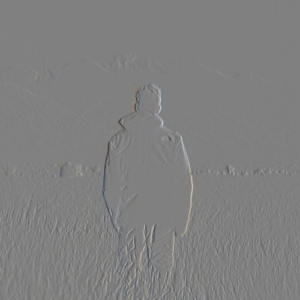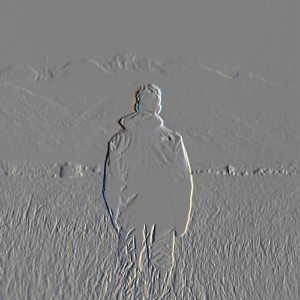Open in App
Not now

# Wand image.emboss in Python

• Last Updated : 22 Apr, 2020

Emboss effect is similar to Edge extraction but it is more like a 3d effect. The traditional use for Emboss is to make something look more three-dimensional by adding highlights and shadows to different parts of your layer. In order to generate an Embossed image we use emboss function in Wand. Similar to edge() function, emboss() function gives best result with grayscale image.

Syntax :

```wand.image.emboss(radius=radius, sigma=std. deviation)
```

Parameters :

ParameterInput TypeDescription
sigmanumbers.Real

Standard deviation used

Image Used :Example #1:

 `# import Image from wand.image module``from` `wand.image ``import` `Image`` ` `# read image using Image function``with Image(filename ``=``"frameman.jpeg"``) as img:`` ` `    ``# generate a grayscale image``    ``img.transform_colorspace(``'gray'``)`` ` `    ``# GENERATE EMBOSS IMAGE``    ``img.emboss(radius ``=` `3.0``, sigma ``=` `1.75``)`` ` `    ``# SAVE FINAL IMAGE``    ``img.save(filename ``=``"manemboss.jpeg"``)`

Output:Example #2:
 `# import Image from wand.image module``from` `wand.image ``import` `Image`` ` `# read image using Image function``with Image(filename ``=``"frameman.jpeg"``) as img:`` ` `    ``# generate a grayscale image``    ``img.transform_colorspace(``'gray'``)`` ` `    ``# GENERATE EMBOSS IMAGE``    ``img.emboss(radius ``=` `10``, sigma ``=` `3``)`` ` `    ``# SAVE FINAL IMAGE``    ``img.save(filename ``=``"manemboss.jpeg"``)`